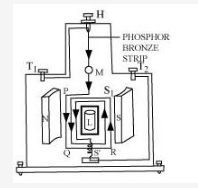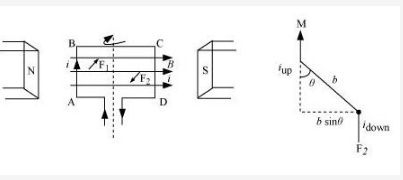# Draw a labelled diagram of a moving coil galvanometer. State the principle on which it works

Draw a labelled diagram of a moving coil galvanometer. State the principle on which it works.

Deduce an expression for the torque acting on a rectangular current carrying loop kept in a uniform magnetic field.Write two factors on which the current sensitivity of a moving coil galvanometer depends.

Principle: A current carrying coil suspended in a magnetic field experiences a torque.Let I be the current through the loop PQRS.

a, b = Sides of the rectangular loop

A = ab = Area of the loop

N = Number of turns in the loopAccording to Fleming’s left hand rule, the side AB experiences a normal inward force, F1= IaB and side CD experiences a normal outward force, F2 = IaB. These two equal and opposite forces form a couple, which exerts a torque given by,= Force × Perpendicular distance

= IaB × b sin= IBab sinτ = IBA sinFor N turns,

τ = NIBA sinThe current sensitivity of a moving coil galvanometer depends on

(i) number of turns in the galvanometer coil
(ii) torsional constant of the suspension fibre.